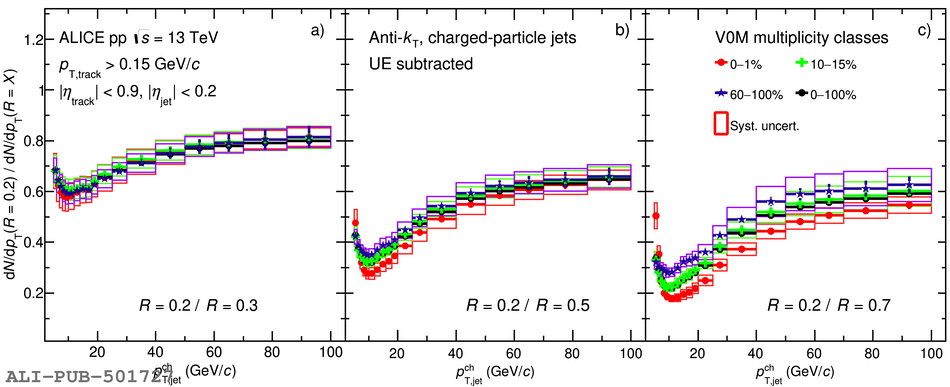# Figure 10

 Comparison of jet spectra ratios of $R = 0.2$ to other radii $R =$ a) $0.3$, b) $0.5$, c) $0.7$ in MB events and in three multiplicity intervals (0--1\%, 10--15\% and 60--100\%). Statistical and systematic uncertainties are shown as vertical error bars and boxes around the data points, respectively. Results for other radii can be found in Appendix Fig. \ref{fig:MultJetCSRatioCompData02046}.